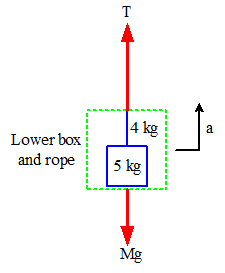# Two masses connected by a weighted rope pulled vertically

## Homework Statement

Two masses are connected by a heavy uniform rope (mass = 4 kg) and a upward force of 200N (Fa) is applied to the block on top; essentially the two masses and the rope are pulled up vertically. The mass on top (m1) weighs 6 kg, the mass on the bottom (m2) weighs 5 kg.

200 N

|
m1
|
| ← 4.0 kg rope
|
m2

Find the tension at the top and middle of the heavy rope.

## Homework Equations

Newton's Second Law, Fnet = ma
Force of gravity, mg

## The Attempt at a Solution

I found the acceleration of the whole system:
Fnet = ma
Fg + Fa = (6+4+5)(a)
a = 3.52 m/s^2 [upwards]

So for the top of the rope I figured that the tension should be supporting the weight of the the mass below it, Fg = mg = (4+5)(9.81), but I also know that since the system is accelerating upwards the tension should be greater, so:

Fnet = Force pulling the rope up + Force of gravity + Tension = (mass of rope + mass of m2)(g)

But I'm not really sure if the magnitude of the force pulling the rope up is 200N, and how I would find it if it isn't.

I have the same problem considering the tension in the middle
Here's my Fnet for that:

Fnet = Tension pulling it up + Tension pulling it down + Fg = (mass of half the rope + mass of m2)(a)

I think I have just a big lack of understanding of tension and I'm confused about what to do... any help /clarification about what path I should take would be greatly appreciated :)

gneill
Mentor
Hi Owen443, Welcome to Physics Forums.

You've calculated the overall acceleration of the system properly, so that's good.

To determine the tension at the top of the rope, imagine that you were to replace the rope and lower box with an equivalent mass and draw a free body diagram for that mass. You know the acceleration of that mass so what can you say about the net force acting on that mass? What forces have to add up to that net force?

Thanks for the quick reply and welcome :)

If I consider the rope and the lower box as a single mass, I can find that the net force acting on it is Fnet = ma = (4+5)(3.52) = 31.68 N [upwards]. The problem I'm having is that I'm not 100% sure what forces have to add up to that net force. The way I see it now is that:

A. The upward force is the force applied by m1 (which I don't think is 200N), and the downward force is that of gravity

B. Upward force is force applied by m1 and the downward force is tension, but I know neither value for both, just that their vector sum is = 31.68 [upwards]

If I consider the midway point of the rope, I'd imagine that it'd be replaced with an equivalent mass (equal to half the mass of the rope and the mass of the lower box), but wouldn't the two forces acting on the FBD both be tension? I'm just having trouble identifying the exact forces acting on the points for each mass :(

gneill
Mentor
You're almost there. You've identified two of the forces that you can find values for: The force due to gravity on the mass and the net force that accelerates it. The unknown tension at the top of the rope is the upward force applied. Three forces only one of which is unknown...#### Attachments

Ah thanks so much, I think I get it now :)

So if considering the top of the rope (where up is + and down is -)
##\vec F_{net} = \vec T + \vec F_g = m\vec a##
##\vec T - (4+5)|\vec g| = (4+5)(3.52)##
##\vec T = 120 N##

And if considering the middle of the rope:
##\vec F_{net} = \vec T + \vec F_g = m\vec a##
##\vec T - (2+5)|\vec g| = (2+5)(3.52)##
##\vec T = 93.3 N##

Am I correct here? And again thank you so much, really appreciate it :)

haruspex
Homework Helper
Gold Member
2020 Award
Ah thanks so much, I think I get it now :)

So if considering the top of the rope (where up is + and down is -)
##\vec F_{net} = \vec T + \vec F_g = m\vec a##
##\vec T - (4+5)|\vec g| = (4+5)(3.52)##
##\vec T = 120 N##

And if considering the middle of the rope:
##\vec F_{net} = \vec T + \vec F_g = m\vec a##
##\vec T - (2+5)|\vec g| = (2+5)(3.52)##
##\vec T = 93.3 N##

Am I correct here? And again thank you so much, really appreciate it :)
That all looks right.